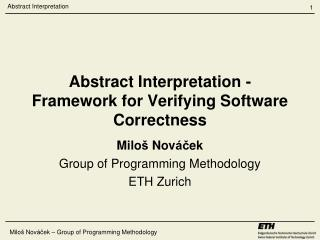# Abstract Interpretation - Framework for Verifying Software Correctness - PowerPoint PPT PresentationDownload PresentationAbstract Interpretation - Framework for Verifying Software Correctness

Abstract Interpretation - Framework for Verifying Software CorrectnessDownload Presentation## Abstract Interpretation - Framework for Verifying Software Correctness

- - - - - - - - - - - - - - - - - - - - - - - - - - - E N D - - - - - - - - - - - - - - - - - - - - - - - - - - -
##### Presentation Transcript

1. Miloš Nováček – Group of Programming Methodology Abstract Interpretation - Framework for Verifying Software Correctness Miloš Nováček Group of Programming Methodology ETH Zurich

2. Miloš Nováček – Group of Programming Methodology Happened to all of us

3. Miloš Nováček – Group of Programming Methodology

4. Miloš Nováček – Group of Programming Methodology Software verification // PRE: true // POST: result ≥ 0 • int abs(int n) { • int result; • if (n<0) { • result = n*(-1); • } else { • result = n; • } • return result; • } Term covering a collection of methods for proving or disproving a software correctness with respect to a given specification

5. Miloš Nováček – Group of Programming Methodology Control flow graph n on the input int result n < 0 false true result = n result = -n return result

6. Miloš Nováček – Group of Programming Methodology Software verification (cont’d) If the intersection is empty, then the program is correct

7. Miloš Nováček – Group of Programming Methodology Formal verification vs Testing Verification Testing Can only detect presence of errors but not their absence Can not find all the errors Easily automatized Widely used – much cheaper than verification Does not require high mathematical skill • Mathematical proof of absence of errors in a program w.r.t. specifications • Expensive and requires high qualification • Limited automatization

8. Miloš Nováček – Group of Programming Methodology Concrete semantics • A program denotes computations in some universe of objects called a concrete domain. (E.g. program states that record the integer value of every program variable) • Concrete semantics of a programming describes these computations. • A collecting interpreter gathers semantic information about a program. However, this information is in general not computable.

9. Miloš Nováček – Group of Programming Methodology Formal software verification is hard Undecidabilityand algorithmic complexity issues are the main obstacles to application of local analysis techniques.

10. Miloš Nováček – Group of Programming Methodology Abstract domain and semantics • Not all the semantic information is required for a specific verification task • Only the necessary information can by abstracted into an abstract domain • Computations on an abstract domain are described by an abstract semantics

11. Miloš Nováček – Group of Programming Methodology Abstract Interpretation “A program denotes computations in some universe of objects. Abstract interpretation of programs consists in using that denotation to describe computations in another universe of abstract objects, so that the results of abstract execution give some informationson the actual computations.” P. & R. Cousot

12. Miloš Nováček – Group of Programming Methodology Abstract interpretation (cont’d) What are we trying to do? Approximate an uncomputable concrete semantics with a computable abstract one. How do we do this? We define the semantics of a program as the fixpointof a monotonic function.

13. Miloš Nováček – Group of Programming Methodology Galois connection Let <C,≤> be a poset that represents a concrete domain and <A,≤> be a poset that represents an abstract domain. Then functions α : C → A and γ : A → C form a Galois connection iff α(c) ≤ a ↔ γ(a) ≤ c. For abstract interpretation to be sound, each pair of abstraction and concretization functions must form a Galois connection.

14. Miloš Nováček – Group of Programming Methodology Sign domain – non-relational All the integers T - + Negative integers Non-negative integers ┴ Empty set of integers

15. Miloš Nováček – Group of Programming Methodology Sign domain - multiplication

16. Miloš Nováček – Group of Programming Methodology Control flow graph n = T int result n = T result = T n = + result = T n < 0 n = - result = T false true result = n result = -n n = + result = + n = - result = + return result n = T result = +

17. Miloš Nováček – Group of Programming Methodology Convex polyhedra x ≤ y x ≤ 2 0 ≤ y

18. Miloš Nováček – Group of Programming Methodology Polyhedra abstract domain • Relational domain capable of keeping track of numerical relations between program variables • Two representations: constraints and frame (extreme vertices, vectors and lines) • Exponential complexity of computation – Chernikova’s algorithm

19. Miloš Nováček – Group of Programming Methodology only x ≤ y Miloš Nováček – Group of Programming Methodology